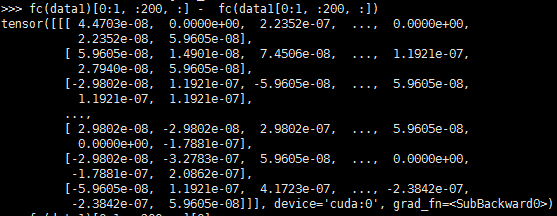# why the result of torch.nn.Linear() isn't consistent when the length of data is different?

for example:
fc = torch.nn.Linear(128,512)
data1 = torch.randn(4,512,128)
data2 = data1[0:1,200,128)#the length of data is changed
result1 = fc(data1)[0:1,200,:]
result2 = fc(data2)
print(result1-result2)#the result1 and result2 should be the same, however they are different in 10^-7

I didn’t find any reason for this result, I hope someone can help me. Thank You.(torch version is 1.1.0)

the result is different in the conditions shown in the following image:Floating point numbers have a limited precision, which results in these small differences.
If you need more precision for whatever reason, you could cast your tensors to `.double()`.
Have a look at this nice blog post about floating point precision.